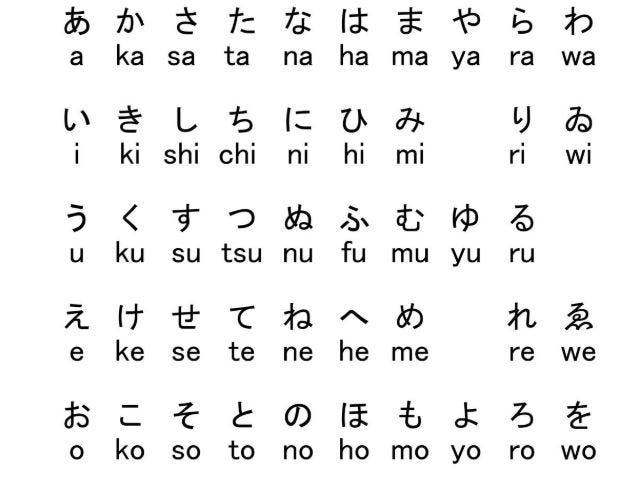# Computer made Japanese letters through Variational Autoencoder

Source: Deep Learning on Medium

It’s been a while since I posted a new blog post having been busy with other things in my life. I have been working on this project for a while now. And now, when it is finally done I can share it with you.

Besides my passion to Machine Learning and AI algorithm in general, I have another not very common hobby, and it is the Japanese language. I have been studying it for a while now and can even get to technical words in ML field (機械学習 and デイープラーニング ) although I still have a long way to go. With this said, I thought to myself, why not join my two biggest passions together and build a cool project?

I decided to design a computer algorithm which can reproduce Japanese letters (especially Hiragana and Katakana – ひらがなとカタカナ) using a Variational autoencoder.

The database I used in this project is from the “ ETL Character Database”. The letters are organized in a very unusual way so make sure to read the instructions of how to handle the different databases (ETL 1–9).

The first part we will cover is the preprocessing of our data. As I said, the database is not very friendly to Data scientists (although of course massive projects are on a whole different caregory). Let’s open the dataset:

`import bitstringimport numpy as npfrom PIL import Image, ImageEnhancefrom PIL import ImageOps, ImageMathfrom matplotlib import pyplot as pltimport cv2%pylab inline`
`t56s = '0123456789[#@:>? ABCDEFGHI&.](<  JKLMNOPQR-\$*);\'|/STUVWXYZ ,%="!'`
`def read_record_ETL4(f, pos=6112):   f = bitstring.ConstBitStream(filename=f)   f.bytepos = pos * 2952   r = f.readlist('2*uint:36,uint:8,pad:28,uint:8,pad:28,4*uint:6,pad:12,15*uint:36,pad:1008,bytes:21888')   return rfilename = 'ETL4/ETL4/ETL4C' # specify the ETL4 filename herer = read_record_ETL4(filename)iF = Image.frombytes('F', (r, r), r[-1], 'bit', 4)iP = iF.convert('L')enhancer = ImageEnhance.Brightness(iP)iE = enhancer.enhance(r)plt.imshow(iE)`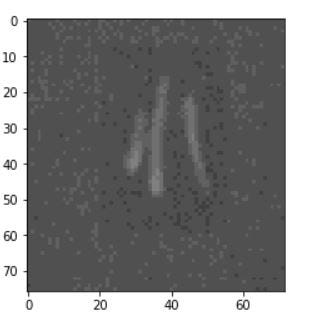Outpt of this piece of code

In this part I am opening a single character from the database (using ETL -4 database only at the moment). The code I am using is taken from here with different tweaks concerning the execution (e.g. bitstring is not compatible with tensorflow in my system, so I had to split to two notebooks, one for preprocessing and the other for the model itself). As you can see the letter needs a serious preprocessing like cropping, filtering out the noise and stronger greyscale contrast in order to recoginze the character (which is 小 by the way, means “small” ).

`def create_data():    data =  np.zeros((6113,76,72))    for i in range(6113):        r = read_record_ETL4(filename,pos=i)        iF = Image.frombytes('F', (r, r), r[-1], 'bit', 4)        iP = iF.convert('L')        enhancer = ImageEnhance.Brightness(iP)        iE = enhancer.enhance(r)        temp=np.array(iE)        data[i,:,:] = temp    return datadata = create_data()`

This function creates the dataset itself in order to handle it in numpy array for convenience. There are 6113 pictures (grey scale) with resolution of 76×72 pixels. Let’s set a function that cleans our dataset.
I implemented a simple gaussian blur and then thresholding (otsu’s histogram method) and a “TOZERO” binarization in order to preserve the stroke pressure grey scale. Did this in order to get a better a results hopefully later on, When we create the Japanese letters.

`def preprocessing_data(data1):    # this function cleans the images and binarize them in order to create a better dataset for our VAE    kernel = np.ones((3,3),np.float32)/9    crop_template = np.zeros((data.shape,data1.shape,data1.shape)) # cropping template    for i in range(data1.shape):          dst = cv2.GaussianBlur(data1[i,:,:],(3,3),0) # smoothing          ret,data1[i,:,:] = cv2.threshold(dst,0,255,cv2.THRESH_TOZERO+cv2.THRESH_OTSU) #binarizing          crop_template[i,:,:] = data1[i,:72,:] # cropping    return crop_templatedata = np.array(data, dtype = np.uint8) # 8 bit unsigned pictures for opencvdata1=data.copy() #copy by value(and not by reference)data1 = preprocessing_data(data1)`

Let’s check some random samples from the dataset.

`ran =np.random.randint(int(data1.shape), size=(2, 1))figure()subplot(1,4,1),imshow(data[int(ran),:,:],cmap = 'gray')title('original  {}'.format(int(ran))), xticks([]), plt.yticks([])subplot(1,4,2),imshow(data1[int(ran),:,:],cmap='gray')title('Sample  {}'.format(int(ran))), xticks([]), plt.yticks([])clim([0, 45])subplot(1,4,3),imshow(data[int(ran),:,:],cmap = 'gray')title('original  {}'.format(int(ran))), xticks([]), plt.yticks([])subplot(1,4,4),imshow(data1[int(ran),:,:],cmap='gray')title('Sample  {}'.format(int(ran))), xticks([]), plt.yticks([])clim([0, 45])`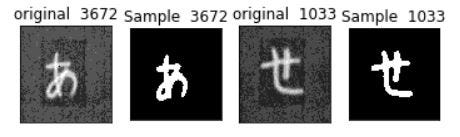Output of our preprocessing

Great, let’s move on to our model after this preprocessing phase.

First, I will explain in a nutshell the concept of the VAE in order to shed some light for those of you who are not familiar with this architecture. For a more in depth and elaborate explanation you can try this page. It gives a very thorough explanation about the relationship with Probablistic graphical models and deep learning concepts.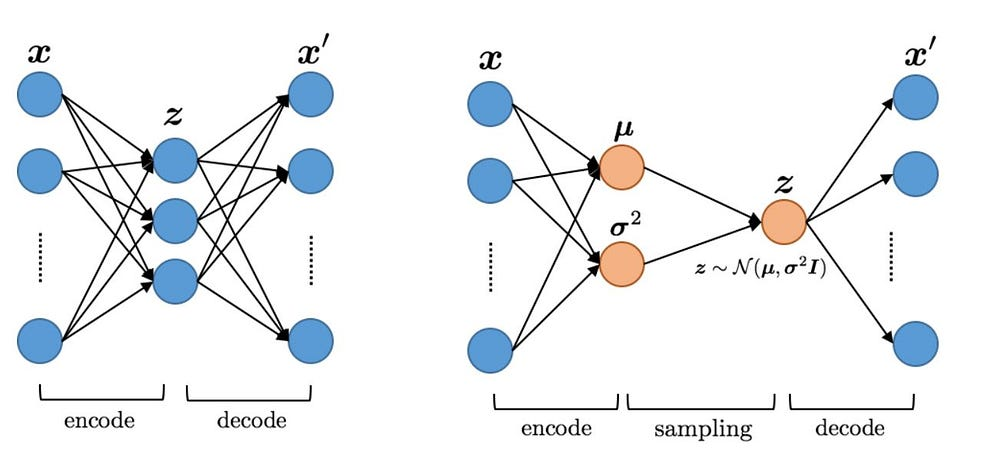Regular autoencoder (right side) and a Variational Autoencoder (left side)

Autoencoders are in great use in many fields of data science. The autoencoders can be used to compression of feature vectors, anomaly detection etc. It is based on unsupervised approach. The main idea of the autoencoder is to encode the data (labeled x in the graph) to a smaller dimension vector and then try to decode it back to the original (reconstruct) x’. The main difference between the AE (Autoencoder) and the VAE is that in the VAE the middle layer is considered as a normal distribution (every node represents its own normal distribution). How do we achieve that? — good question.

2 main things are different then the AE:

1. The loss function used in the model contains two elements: the first is the reconstruction loss which is the same as in the AE in order to train the network to reconstruct the data. and the second element is KL(Kullback -leibler) divergence loss. The KL divergence represents how different two distributions are. It has very unique characteristics like non symmetry, direct relation to fischer information metric and more. We use this loss in order to force the network to capture the layer between the encoder and the decoder to capture distribution similar to normal distribution. The KL divergence works as a regularizer.
2. The second difference is the reparameterization trick. Since the network learn it’s parameters using our trusty old back propagation algorithm, it needs to differntiate the layers. If we’re using sampling (like you can see on the right side of the graph), and we want to take derivate of a function of our sampled variable with respect to our parameter we have a problem since our variable is a random variable. The reparameterization trick will solve this problem (and I urge you to read the links I wrote before!).

So you asking, how will the network will synthesize it’s own Japanese letters then?

What we’re going to do is , after we trained the network on our dataset and made sure we were satisfied with the reconstruction, we will sample variables from standard normal distribution. Later, insert it as the input to the Decoder only and watch the output of the network ,which is basically random since we don’t have a designated input besides random variables sampled from a normal distribution! nice, isn’t it?

So our goal here is to train the network with the dataset we preprocessed beforehand in order to create new handwritten Japanese letters that are not part of the dataset but based on it.

I seperated the preprocessing and the model to two seperate notebooks since the “bitstring” package and tensorflow weren’t compatible for some reason on my rig. Saving the data we preprocessed using:

`np.save('Japanese.npy', data1)`

And went on to the next notebook:

`import numpy as npimport tensorflow as tfimport matplotlib.pyplot as pltimport cv2%matplotlib inline`
`data1 = np.load('Japanese.npy')data1 =data1/160 %normalize pixels`

Let’s move on to build our encoder and our model:

`tf.reset_default_graph()# sess = tf.InteractiveSessionbatch_size = 32X_in = tf.placeholder(dtype=tf.float32, shape=[None, 72, 72], name='X')Y    = tf.placeholder(dtype=tf.float32, shape=[None, 72, 72], name='Y')Y_flat = tf.reshape(Y, shape=[-1, 72 * 72]) #for estimating losskeep_prob = tf.placeholder(dtype=tf.float32, shape=(), name='keep_prob')dec_in_channels = 1n_latent = 12reshaped_dim = [-1, 7, 7, dec_in_channels]inputs_decoder = int(49 * dec_in_channels / 2)def lrelu(x, alpha=0.3):return tf.maximum(x, tf.multiply(x, alpha))`
`def encoder(X_in, keep_prob):    activation = lreluwith tf.variable_scope("encoder", reuse=None):        X = tf.reshape(X_in, shape=[-1, 72, 72, 1])        x = tf.layers.conv2d(X, filters=64, kernel_size=4, strides=2, padding='same', activation=activation)        x = tf.nn.dropout(x, keep_prob)        x = tf.layers.conv2d(x, filters=64, kernel_size=4, strides=2, padding='same', activation=activation)        x = tf.nn.dropout(x, keep_prob)        x = tf.layers.conv2d(x, filters=64, kernel_size=4, strides=1, padding='same', activation=activation)        x = tf.nn.dropout(x, keep_prob)        x = tf.layers.Flatten()(x)        mn = tf.layers.dense(x, units=n_latent)        sd       = 0.5 * tf.layers.dense(x, units=n_latent)                    epsilon = tf.random_normal(tf.stack([tf.shape(x), n_latent]))         z  = mn + tf.multiply(epsilon, tf.exp(sd))return z, mn, sd`

The batch size is set to 32, as a loss function we have a leaky relu (probably other loss functions will do in this simple model) with the negative end slope set to 0.3 (totally arbitrary). Our dataset is made of greyscale images of 72*72 . dropout helped to avoid isolated activated neurons and overfitting. Kernel size not too big of 4 and other standard hyperparameters. The number of latent units (the sampled layer) is 12 after several failed optimization attempts.

z variable holds all the hidden units composed of mean and a standard deviation multiplied by a normal distribution sampled epsilon (This is the reparametrization trick mentined before)

`def decoder(sampled_z, keep_prob):with tf.variable_scope("decoder", reuse=None):        x = tf.layers.dense(sampled_z, units=inputs_decoder, activation=lrelu)        x = tf.layers.dense(x, units=inputs_decoder * 2 + 1, activation=lrelu)        x = tf.reshape(x, reshaped_dim)        x = tf.layers.conv2d_transpose(x, filters=64, kernel_size=4, strides=2, padding='same', activation=tf.nn.relu)        x = tf.nn.dropout(x, keep_prob)        x = tf.layers.conv2d_transpose(x, filters=64, kernel_size=4, strides=1, padding='same', activation=tf.nn.relu)        x = tf.nn.dropout(x, keep_prob)        x = tf.layers.conv2d_transpose(x, filters=64, kernel_size=4, strides=1, padding='same', activation=tf.nn.relu)        x = tf.layers.Flatten()(x)        x = tf.layers.dense(x, units=72*72, activation=tf.nn.sigmoid)        img = tf.reshape(x, shape=[-1, 72, 72])return img`

This is the decoder, it recieves a sampled z like we mentioned before (12 latent variables) and reconstructs the image.

`sess = tf.Session()sampled, mn, sd = encoder(X_in, keep_prob)dec = decoder(sampled, keep_prob)`
`unreshaped = tf.reshape(dec, [-1, 72*72])img_loss = tf.reduce_sum(tf.squared_difference(unreshaped, Y_flat), 1)latent_loss = -0.5 * tf.reduce_sum(1.0 + 2.0 * sd - tf.square(mn) - tf.exp(2.0 * sd), 1)loss = tf.reduce_mean(img_loss + latent_loss ) optimizer = tf.train.AdamOptimizer(0.0005).minimize(loss)sess = tf.Session()sess.run(tf.global_variables_initializer())`

The most important part in this piece of code is the loss function definition, as we discussed before we have two parts, the image loss (MSE/L2 loss for this simple image) and the latent loss for the KL divergence. Adam optimizer, learning rate and all the other stuff are quite standard.

`def next_batch(num, data):'''    Return a total of `num` random samples and labels.     '''    idx = np.arange(0 , len(data))    np.random.shuffle(idx)    idx = idx[:num]    data_shuffle = [data[ i] for i in idx]return np.asarray(data_shuffle)# batch = next_batch(batch_size, data1)`

This is the batch function for convenient input. And now let’s move on to train the network.

`for i in range(30000):    batch = next_batch(batch_size, data1)    sess.run(optimizer, feed_dict = {X_in: batch, Y: batch, keep_prob: 0.5})if not i % 100:        ls, d, i_ls, d_ls, mu, sigm = sess.run([loss, dec, img_loss, latent_loss, mn, sd], feed_dict = {X_in: batch, Y: batch, keep_prob: 1.0})        plt.imshow(np.reshape(batch, [72, 72]), cmap='gray')        plt.show()        plt.imshow(d, cmap='gray')        plt.show()        print('iteration: {}, loss:{}, image loss:{}, distribution loss:{}'.format(i, ls, np.mean(i_ls), np.mean(d_ls)))`

You can see here the reconstruction ability in the beginning of training(left) and the end of the training session (right).

Now, after we finished training let’s see if the decoder is able to produce a new letter from a random sampled z (normally distributed).

`randoms = [np.random.normal(0, 1, n_latent) for _ in range(1)]imgs = sess.run(dec, feed_dict = {sampled: randoms, keep_prob: 1.0})imgs = [np.reshape(imgs[i], [72, 72]) for i in range(len(imgs))]# imgs = np.array(imgs)# imgs.shape# for img in imgs:#     plt.figure(figsize=(1,1))#     plt.axis('off')plt.imshow(imgs, cmap='gray')`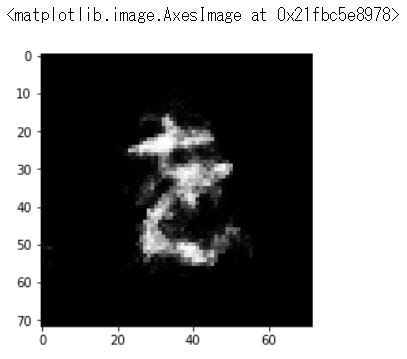End result

Well we got a decent looking さ(“sa”) as you can see. I guess with more hyperparameters tuning we can get way better results.

The notebooks: Preprocessing , Model.

Thanks for reading so far, make sure to take a look at the reference link since I took some of my code from there.

References:

For any questions , let me know: tomer@nahshoh.net

Thank you!